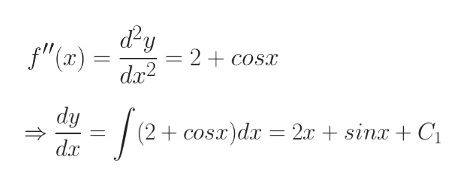Question
11 views

Find f if f''(x)=2+cos(x), f(0)=−2, f(pi/2)=6

f(x)= ?

check_circle

Step 1

Please see the white board. C1 is the constant of integration.help_outlineImage Transcriptionclosedy = 2 + cosx " (ar)da - e+ dy cosx)dx = 2x + sinx + C (2 dx fullscreen
Step 2

We need to integration the expression one more time to get the final answer. Please see the white board.

Step 3

When x = 0; f(0) = -2

Hence, 02 - cos0 + C1 x 0 + C2 = C2 - 1 = -2

Hence, C2 = 1 - 2 = -1

Hence, f(x...

### Want to see the full answer?

See Solution

#### Want to see this answer and more?

Solutions are written by subject experts who are available 24/7. Questions are typically answered within 1 hour.*

See Solution
*Response times may vary by subject and question.
Tagged in
MathCalculus

### Differential Equations